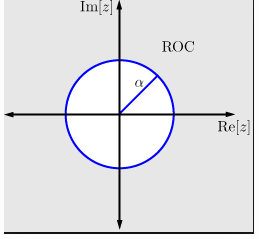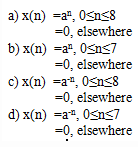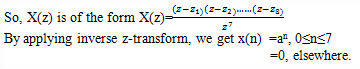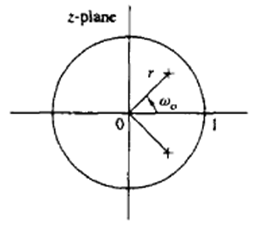Test: Rational Z Transform

# Test: Rational Z Transform

Test Description

## 10 Questions MCQ Test Digital Signal Processing | Test: Rational Z Transform

Test: Rational Z Transform for Electrical Engineering (EE) 2022 is part of Digital Signal Processing preparation. The Test: Rational Z Transform questions and answers have been prepared according to the Electrical Engineering (EE) exam syllabus.The Test: Rational Z Transform MCQs are made for Electrical Engineering (EE) 2022 Exam. Find important definitions, questions, notes, meanings, examples, exercises, MCQs and online tests for Test: Rational Z Transform below.
Solutions of Test: Rational Z Transform questions in English are available as part of our Digital Signal Processing for Electrical Engineering (EE) & Test: Rational Z Transform solutions in Hindi for Digital Signal Processing course. Download more important topics, notes, lectures and mock test series for Electrical Engineering (EE) Exam by signing up for free. Attempt Test: Rational Z Transform | 10 questions in 10 minutes | Mock test for Electrical Engineering (EE) preparation | Free important questions MCQ to study Digital Signal Processing for Electrical Engineering (EE) Exam | Download free PDF with solutions
 1 Crore+ students have signed up on EduRev. Have you?
Test: Rational Z Transform - Question 1

### What are the values of z for which the value of X(z)=0?

Detailed Solution for Test: Rational Z Transform - Question 1

Explanation: For a rational z-transform X(z) to be zero, the numerator of X(z) is zero and the solutions of the numerator are called as ‘zeros’ of X(z).

Test: Rational Z Transform - Question 2

### What are the values of z for which the value of X(z)=∞?

Detailed Solution for Test: Rational Z Transform - Question 2

Explanation: For a rational z-transform X(z) to be infinity, the denominator of X(z) is zero and the solutions of the denominator are called as ‘poles’ of X(z).

Test: Rational Z Transform - Question 3

### If X(z) has M finite zeros and N finite poles, then which of the following condition is true?

Detailed Solution for Test: Rational Z Transform - Question 3

Explanation: If X(z) has M finite zeros and N finite poles, then X(z) can be rewritten as X(z)=z -M+N.X'(z).
So, if N>M then z has a positive power. So, it has |N-M| zeros at origin.

Test: Rational Z Transform - Question 4

If X(z) has M finite zeros and N finite poles, then which of the following condition is true?

Detailed Solution for Test: Rational Z Transform - Question 4

Explanation: If X(z) has M finite zeros and N finite poles, then X(z) can be rewritten as X(z)=z-M+N.X'(z).
So, if N < M then z has a negative power. So, it has |N-M| poles at origin.

Test: Rational Z Transform - Question 5

Which of the following signals have a pole-zero plot as shown below?Detailed Solution for Test: Rational Z Transform - Question 5

Explanation: From the given pole-zero plot, the z-transform of the signal has one zero at z=0 and one pole at z=a.
So, we obtain X(z)=z/(z-a)
By applying inverse z-transform for X(z), we get
x(n)= anu(n).

Test: Rational Z Transform - Question 6

Which of the following signals have a pole-zero plot as shown below?(Let M=8 in the figure)Detailed Solution for Test: Rational Z Transform - Question 6

Explanation: From the figure given, the z-transform of the signal has 8 zeros on circle of radius ‘a’ and 7 poles at origin.Test: Rational Z Transform - Question 7

The z-transform X(z) of the signal x(n)=anu(n) has:

Detailed Solution for Test: Rational Z Transform - Question 7

Explanation: The z-transform of the given signal is X(z)= z/(z-a)
So, it has one pole at z=a and one zero at z=0.

Test: Rational Z Transform - Question 8

What is the nature of the signal whose pole-zero plot is as shown?Detailed Solution for Test: Rational Z Transform - Question 8

Explanation: From the pole-zero plot, it is shown that r < 1, so the signal is a decaying signal.

Test: Rational Z Transform - Question 9

What are the values of z for which the value of X(z)=0?

Detailed Solution for Test: Rational Z Transform - Question 9

Explanation: For a rational z-transform X(z) to be zero, the numerator of X(z) is zero and the solutions of the numerator are called as ‘zeros’ of X(z).

Test: Rational Z Transform - Question 10

If Y(z) is the z-transform of the output function, X(z) is the z-transform of the input function and H(z) is the z-transform of system function of the LTI system, then H(z)=?

Detailed Solution for Test: Rational Z Transform - Question 10

Explanation: We know that for an LTI system, y(n)=h(n)*x(n)
On applying z-transform on both sides we get, Y(z)=H(z).X(z)=>H(z)= ( Y(z))/(X(z) ).

## Digital Signal Processing

3 videos|50 docs|54 tests
 Use Code STAYHOME200 and get INR 200 additional OFF Use Coupon Code
Information about Test: Rational Z Transform Page
In this test you can find the Exam questions for Test: Rational Z Transform solved & explained in the simplest way possible. Besides giving Questions and answers for Test: Rational Z Transform, EduRev gives you an ample number of Online tests for practice

## Digital Signal Processing

3 videos|50 docs|54 tests